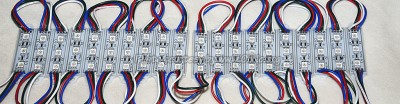# OliNo

Renewable Energy

## Duxoled – Led module DUX12-RGB3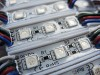presents an LED module. The measurements from OliNo show that the lamp consumes 0.7 W and has a luminous flux of 22 lm. This results in an efficacy of 30 lm/W. The lamps energy category label is A+.

Note: one lamp has been measured on light output while more lamps were connected on the power supply (in this case 20.0 pieces). This to make the measurement more accurate. All power and current values of the total set are computed back to one lamp.

This article shows the measurement results. Many parameters are also found in the Eulumdat file.

See this overview for a comparison with other light bulbs.

### Summary measurement data

parameter meas. result remark
Luminous intensity Iv 6.8 Cd Measured straight underneath the lamp.
Illuminance modulation index 0 % Measured with a light sensor looking at the lamp (angle not defined). Is a measure for the amount of flickering.
Beam angle 128 deg 128 deg is the beam angle for the C0-C180-plane (perpendicular to the length direction of the lamp) and 125 deg is the beam angle for the C90-C270 plane, which is along the length direction of the lamp.
Power P 0.7 W The net power consumed.
Power Factor 1.00 The tests were done with a DC power supply. This results in no blind power and as a result the power factor is always 1.0 but not relevant to mention.
THD NaN % Total Harmonic Distortion, is not present as a DC voltage was used to power the lamp so a DC current resulted which has no THD.
Luminous flux 22 lm Measured with photogoniometer, calculation done as described in LM79-08.
Luminous efficacy 30 lm/W Be aware that a DC power supply has been used. The found efficacy with this measurement is excluding the power supply that normally is needed to convert the grid voltage (230 V AC) to the used DC voltage. By excluding the consumption of the power supply the efficacy found here is higher than it would be when the power supply had been included.
EU2013-label classification A+ The energy class, from A++ (more efficient) to E (least efficient). This label is an update of the previous version, and compulsory from Sept 2013.
CRI_Ra 2 Color Rendering Index.
CQS 26.0 QCS (v9.0.3) is an improved indicator (over CRI) of how well colors are rendered.
Coordinates chromaticity diagram x=0.2246 en y=0.2712
Fitting 12V DC This module is connected to 12V DC.
PAR-value 0.1 uMol/s/m2 The number of photons seen by an average plant when it is lit by the light of this light bulb. Value valid at 1 m distance from light bulb.
PAR-photon efficacy 0.5 uMol/s/We The total emitted number of photons by this light, divided by its consumption in W. It indicates a kind of efficacy in generating photons.
Photon current 0.6 uMol/s The total number of photons in the light of this lamp.
S/P ratio 4.1 This factor indicates the amount of times more efficient the light of this light bulb is perceived under scotopic circumstances (low environmental light level).
L x W x H external dimensions 61 mm x 15 mm x 6 mm External dimensions of the lamp.
L x W luminous area 15 mm x 5 mm Dimensions of the luminous area (used in Eulumdat file). It is the surface of the LEDs.
General remarks The ambient temperature during the whole set of illuminance measurements was 26.0 – 26.1 deg C.The temperature of the lamp gets maximally about 78 degrees hotter than ambient temperature.Warm up effect: During the warmup time the illuminance varies during 19 minutes and decreases with 10 %.
During the warmup time the power doesn’t vary significantly (< 5 %).
The variation in efficacy (calculated as indication by simplydividing the illuminance by the power) during the warming up is -13 %. A very high negative value indicates a significant decrease for instance due to heating up of the lamp (decrease of lifetime).Voltage dependency: There is a constant dependency of the illuminance when the power voltage varies between 10 – 14 V DC.
There is a constant dependency of the consumed power when the power voltage varies between 10 – 14 V DC.At the end of the article an additional photo.
Eff-variation -13 % This is the variation in efficacy (calculated as indication by simply dividing the illuminance by the power) during the warming up. A very high negative value indicates a significant decrease for instance due to heating up of the lamp (decrease of lifetime).
Dimmable no Info from manufacturer.

Biologic effect factor
1.787 According to pre-norm DIN V 5031-100:2009-06.
Blue Light Hazard risk group 1 0=exempt, 1=low, 2 = moderate, 3=high risk.
form factor module
article number DUX12-RGB3
Eulumdat fileRight click on icon and save the file.
IES fileRight click on icon and save the file.
Eulumdat file redRight click on icon and save the file.
Eulumdat file greenRight click on icon and save the file.
Eulumdat file blueRight click on icon and save the file.

Note: one lamp has been measured on light output while more lamps were connected on the power supply (in this case 20.0 pieces). This to represent the most occurring situation. All power and current values of the total set are computed back to one lamp.

### Overview tablePlease note that this overview table makes use of calculations, use this data with care as explained on the OliNo site. E (lux) values are not accurate, when within 5 x 16 mm (maximal luminous size, eventually diagonally measured)= 80 mm. Within this distance from the lamp (data given in red), the measured lux values will be less than the computed values in this overview as the measurements are then within the near field of the lamp.

### EU 2013 Energy label classification

Since Sept 2013 these labels will be needed.

Important for the energy classification are the corrected rated power and the useful luminous flux.
The measured rated power is 0.7 W and might need to be corrected. The correction is dependent from the lamp type and whether or not the lamp control gear is included or not. The choice for this lamp is the following classification: Lamps operating on external LED lamp control gear. As a result the corrected rated power becomes: 0.8 W.
The luminous flux measured is 22 lm. The classification of this lamp needed to determine the useful flux is: Non-directional lamps. Then the useful flux becomes 22 lm. Now a reference power can be calculated.

The energy efficiency coefficient is P_corr / P_ref = 0.16.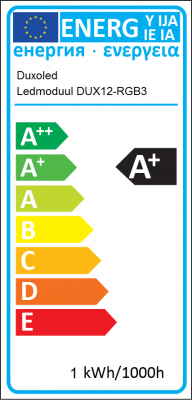EU energy label for this lampZip file with 6 EU energy labels of this lamp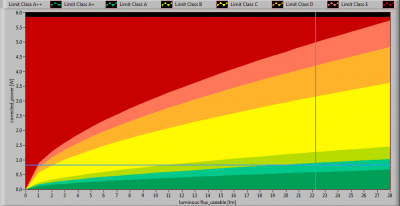The lamp’s performance in the lumen-Watt field, with the energy efficacy fields indicated.

### Eulumdat light diagram

This light diagram below comes from the program Qlumedit, that extracts these diagrams from an Eulumdat file.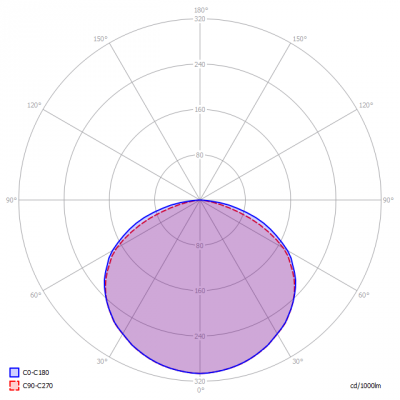The light diagram giving the radiation pattern.

The light diagram indicates the beam in the C0-C180 plane (perpendicular to the length direction of the lamp) and in the plane perpendicular to that, the C90-C270 plane (along the length direction of the lamp).

### Illuminance Ev at 1 m distance, or luminous intensity Iv

Herewith the plot of the averaged luminous intensity Iv as a function of the inclination angle with the light bulb.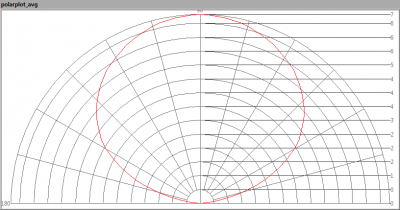The radiation pattern of the light bulb.

This radiation pattern is the average of the light output of the light diagram given earlier. Also, in this graph the luminous intensity is given in Cd.

These averaged values are used (later) to compute the lumen output.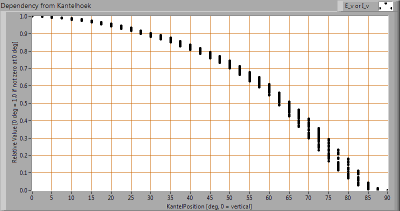Intensity data of every measured turn angle at each inclination angle.

This plot shows per inclination angle the intensity measurement results for each turn angle at that inclination angle. There normally are differences in illuminance values for different turn angles. However for further calculations the averaged values will be used.

When using the average values per inclination angle, the beam angle can be computed, being 128 deg for the C0-C180 plane and 125 deg for the C90-C270 plane.

### Luminous flux

With the averaged illuminance data at 1 m distance, taken from the graph showing the averaged radiation pattern, it is possible to compute the luminous flux.

The result of this computation for this light spot is a luminous flux of 22 lm.

### Luminous efficacy

The luminous flux being 22 lm, and the consumed power of the lamp being 0.7 Watt, results in a luminous efficacy of 30 lm/Watt.

### Electrical properties

The power factor is 1.00. The tests were done with a DC power supply. This results in no blind power and as a result the power factor is always 1.0 but not relevant to mention.

 Lamp voltage 11.98 V Lamp current 0.062 A Power P 0.7 W Apparent power S 0.7 VA Power factor 1.00

### Temperature measurements lamp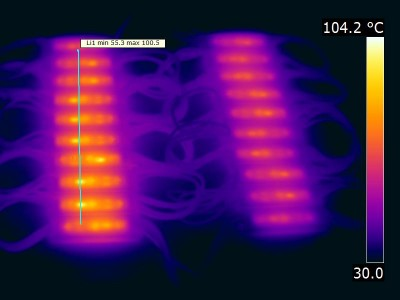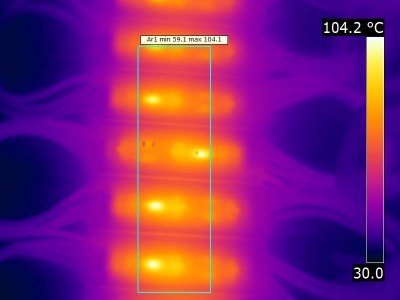Temperature image(s).

 status lamp > 2 hours on ambient temperature 26 deg C reflected background temperature 26 deg C camera Flir T335 emissivity 0.95 measurement distance 0.5, 1 m IFOVgeometric 0.136 mm per 0.1 m distance NETD (thermal sensitivity) 50 mK

### Spectral power distributionThe spectral power distribution of this light bulb, energies on y-axis valid at 1 m distance.

### PAR value and PAR spectrum

To make a statement how well the light of this light bulb is for growing plants, the PAR-area needs to be determined.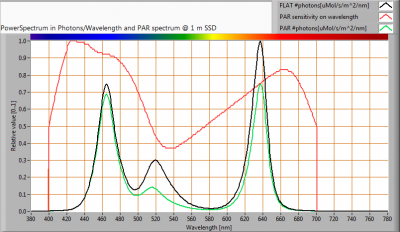The photon spectrum, then the sensitivity curve and as result the final PAR spectrum of the light of this light bulb

parameter value unit
PAR number 0.1 uMol/s/m^2
PAR photon current 0.4 uMol/s
PAR photon efficacy 0.5 uMol/s/W

The PAR efficiency is 75 % (valid for the PAR wave length range of 400 – 700 nm). This is the maximum percentage of the total of photons in the light that is effectively used by the average plant (since the plant might not take 100 % of the photons at the frequency where its relative sensitivity is 100 %).

### S/P ratio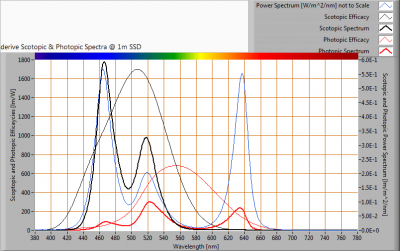The power spectrum, sensitivity curves and resulting scotopic and photopic spectra (spectra energy content defined at 1 m distance).

The S/P ratio of the light coming from this lamp is 4.1.

### Chromaticity diagramThe chromaticity space and the position of the lamp’s color coordinates in it.

The point of the light in this diagram is outside the area indicated with class A. This area indicates an area for signal lamps.

The color coordinates are x=0.2246 and y=0.2712.

### Color Rendering Index (CRI) or also Ra

Herewith the image showing the CRI as well as how well different colors are represented (rendered). The higher the number, the better the resemblance with the color when a black body radiator would have been used (the sun, or an incandescent lamp)

Each color has an index Rx, and the first 8 indexes (R1 .. R8) are averaged to compute the Ra which is equivalent to the CRI.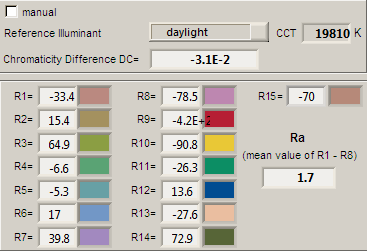CRI of the light of this lightbulb.

This value of 2 indicates how well the light of this lamp can render well a set of reference colors, this in comparison with the light of a reference source (for color temperatures < 5000K a black radiator is used as reference and for color temperatures > 5000K the sun or the light outside during the day).

The value of 2 is smaller than the value of 80 that is considered as a minimum for working areas in general.

Note: the chromaticity difference is -0.0307 and indicates the distance to the Planckian Locus. There is a value mentioned of max 5.4E-3 in section 5.3 of CIE 13.3-1995 however not further explanation of it.
An other reference with signal lights as a reference is given in the chromaticity diagram.

### Color Quality Scale

QCS (v9.0.3) is an improved indicator (over CRI) of how well colors are rendered.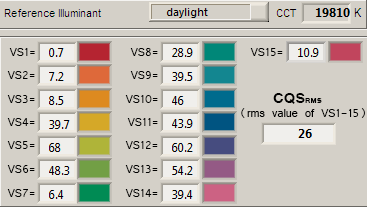CQS-values of the light of this light bulb.CQS-values for the light of this light bulb compared with those of a reference source with the same color temperature.

### Voltage dependency

The dependency of a number of lamp parameters on the lamp voltage is determined. For this, the lamp voltage has been varied and its effect on the following light bulb parameters measured: illuminance E_v [lx], the lamppower P [W] and the luminous efficacy [lm/W] (this latter is estimated here by dividing the found E_v value by P).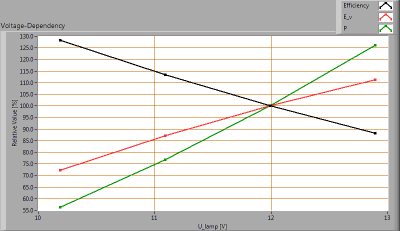Lamp voltage dependencies of certain light bulb parameters

There is a constant dependency of the illuminance when the power voltage varies between 10 – 14 V DC.
There is a constant dependency of the consumed power when the power voltage varies between 10 – 14 V DC.

When the voltage varies abruptly with + or – 0.25 V DC then this results in a variation of the illuminance of maximally 3.6 %. This difference in illuminance is not visible (when it occurs abruptly).

### Warm up effects

After switch on of a cold lamp, the effect of heating up of the lamp is measured on illuminance E_v [lx], the lamppower P [W] and the luminous efficacy [lm/W].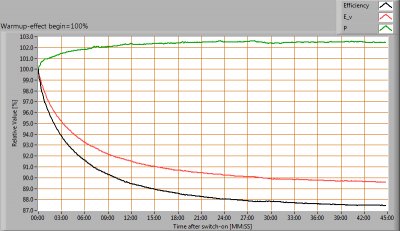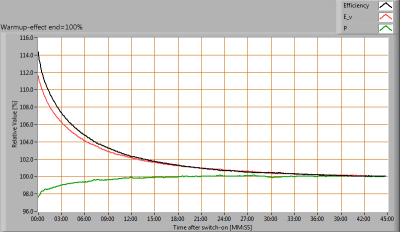Effect of warming up on different light bulb parameters. In the first graph the 100 % level is put at begin, and in the last graph the 100 % level is put at the end.

During the warmup time the illuminance varies during 19 minutes and decreases with 10 %.
During the warmup time the power doesn’t vary significantly (< 5 %).
The variation in efficacy (calculated as indication by simplydividing the illuminance by the power) during the warming up is -13 %. A very high negative value indicates a significant decrease for instance due to heating up of the lamp (decrease of lifetime).

### Measure of flickering

An analysis is done on the measure of flickering of the light output by this light bulb.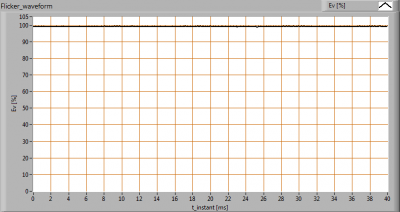The measure of fast illuminance variation of the light of the light bulb

parameter value unit
Flicker frequency 3506.3 Hz
Illuminance modulation index 0 %

The illuminance modulation index is computed as: (max_Ev – min_Ev) / (max_Ev + min_Ev).

### Biologic effect

The biologic effect shows the level of impact the light of this lamp can have on the day-night rhythm of human beings (as well as the suppression of melatonin production).
The important parameters (according to prenorm DIN V 5031-100:2009-06):

 biologic effect factor 1.787 kbiol trans (25 years) 1 kbiol trans (50 years) 0.754 kbiol trans (75 years) 0.48 kpupil(25 years) 1 kpupil(50 years) 0.74 kpupil(75 years) 0.519

### Blue Light Hazard

The amount of blue light and the harm it can cause on the retina has been determined. Herewith the results.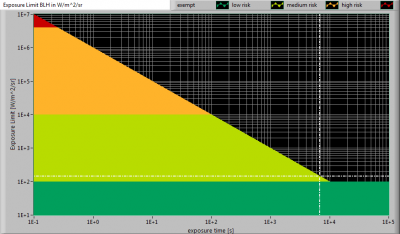The level of blue light of this lamp related to the exposure limit and the different classification areas.

 L_lum0 [mm] 5 Dimension of brightest part of lamp in C0-C180 direction. L_lum90 [mm] 15 Dimension of brightest part of lamp in C90-C270 direction. SSD_500lx [mm] 117 Calculated distance where Ev = 500 lux. This computation is valid when it is in the far field of the lamp. Note: if this value 200 mm then the distance of 200 mm is taken as proposed in the norm IEC 62471:2006. Start of far field [mm] 79 Minimum distance at which the lamp can be seen as apoint source. In this area the Ev is linearly dependent from (1/distance)2. 300-350 nm values stuffed with 0s yes In the event OliNo has measured with a SpB1211 spectrometer without UV option then the irradiance data of 300-349 nm is missing. For lamps where there is already no energy content near 350 nm, the values 300-349 can also be set at zero then. alphaC0-C180 [rad] 0.025 (Apparent) source angle in C0-C180 direction. alphaC90-C270 [rad] 0.075 (Apparent) source angle in C90-C270 direction. alphaAVG [rad] 0.050 Average (apparent) source angle. If average >= 0.011 rad then the exposure limit is computed with radiance Lb. Otherwise with irradiance Eb. Exposure value [W/m^2/sr] 1.45E+2 Blue Light Hazard value for this lamp, measured straight underneath the lamp. Computation is referenced to Lb. Blue Light Hazard risk group 1 0=exempt, 1=low, 2 = moderate, 3=high risk.

### Extra